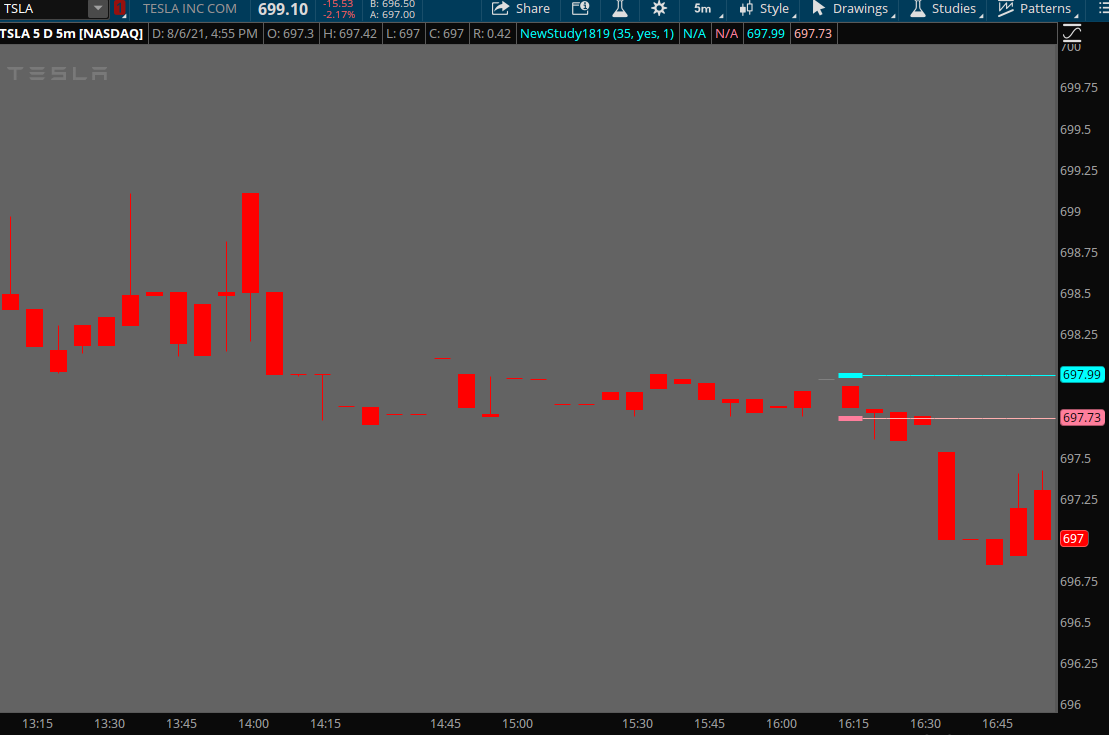# Extend Target lines

#### Billy101

##### New member
Hi Guys,
I've been working on a trend indicator similar to supertrend.
I'm having troubles to extend target lines over time until price crosses.
Thanks!

Code:
``````input lenghtCCI = 35;

input show = yes;

def tgt = 37;

def a = CCI(lenghtCCI)<-tgt;

def b = CCI(lenghtCCI)> tgt;

def state = if a>b then -1 else if a==b then 0 else 1;

AssignPriceColor ( if show == yes then if state == 0 then Color.GRAY else if state == 1 then Color.Blue else Color.RED else color.CURRENT);

def o = open;

def c = close;

def h = high;

def l = low;

def BarLngth = h - l;

def TQRH = h + (BarLngth/2);

def TQRL = l - (BarLngth/2);

def tqron=0;

def statechkh = if state== 0 and state== 1 then TQRH else if state== 0 and state== -1 then TQRH  else double.nan ;

def statechkl = if state== 0 and state== 1 then TQRL else if state== 0 and state== -1 then TQRL else double.nan;

plot TQRTGTH = statechkh ;

TQRTGTH.SetPaintingStrategy(PaintingStrategy.Horizontal);

plot TQRTGTL = statechkl ;

TQRTGTL.SetPaintingStrategy(PaintingStrategy.Horizontal);``````

Last edited by a moderator:

#### horserider

##### Well-known member
VIP
Try adding something like this to the TQRTGTL

Code:
``````def countt = if IsNaN(TQRTGTL) and !IsNaN(TQRTGTL) then 1 else countt + 1;

plot tqrtgltext = if IsNaN(tqrtgtl) then GetValue(tQRTGTL, countt) else Double.NaN;

tqrtgltext.SetPaintingStrategy(PaintingStrategy.HORIZONTAL);

tqrtgltext.SetDefaultColor(Color.PINK);``````

Then repeat for TQRTGTH and of course you may not want pink as the color

•#### Kai

##### New member
Thanks, I used this trick to extend a constant value to the right

#### SleepyZ

##### Well-known member
VIP
Hi Rad, as Saylar says, it comes from Advanced Get ... I need help if you want ... Is there any way to eliminate the previous plots in the code of this topic ... That is, just keep the lines at the end until the candle to change color, and then plot again. I do not know if I explain myself.
I have tried Today / getday / getlastday, but the next day the line distances itself from the candle
See if this helps. Input how many plots you wish at 'plot_limit'Ruby:
``````input lenghtCCI = 35;

input show = yes;

def tgt = 37;

def a = CCI(lenghtCCI) < -tgt;

def b = CCI(lenghtCCI) > tgt;

def state = if a > b then -1 else if a == b then 0 else 1;

AssignPriceColor ( if show == yes then if state == 0 then Color.GRAY else if state == 1 then Color.BLUE else Color.RED else Color.CURRENT);

def o = open;

def c = close;

def h = high;

def l = low;

def BarLngth = h - l;

def TQRH = h + (BarLngth / 2);

def TQRL = l - (BarLngth / 2);

def tqron = 0;

def statechkh = if state == 0 and state == 1 then TQRH else if state == 0 and state == -1 then TQRH  else Double.NaN ;

def statechkl = if state == 0 and state == 1 then TQRL else if state == 0 and state == -1 then TQRL else Double.NaN;

input plot_limit = 1;
def cond = statechkh or statechkl;
rec dataCount = CompoundValue(1, if !IsNaN(cond) then dataCount + 1 else dataCount, 0);

plot TQRTGTH = if HighestAll(dataCount) - dataCount <= plot_limit - 1 then statechkh else Double.NaN;
TQRTGTH.SetPaintingStrategy(PaintingStrategy.HORIZONTAL);

plot TQRTGTL = if HighestAll(dataCount) - dataCount <= plot_limit - 1 then statechkl else Double.NaN;
TQRTGTL.SetPaintingStrategy(PaintingStrategy.HORIZONTAL);

def counth = if IsNaN(TQRTGTL) and !IsNaN(TQRTGTL) then 1 else counth + 1;

plot tqrtghtext = if IsNaN(TQRTGTL) then GetValue(TQRTGTH, counth) else Double.NaN;

tqrtghtext.SetPaintingStrategy(PaintingStrategy.HORIZONTAL);

tqrtghtext.SetDefaultColor(Color.CYAN);

def countl = if IsNaN(TQRTGTL) and !IsNaN(TQRTGTL) then 1 else countl + 1;

plot tqrtgltext = if IsNaN(TQRTGTL) then GetValue(TQRTGTL, countl) else Double.NaN;

tqrtgltext.SetPaintingStrategy(PaintingStrategy.HORIZONTAL);

tqrtgltext.SetDefaultColor(Color.PINK);``````

### Not the exact question you're looking for?

87k+ Posts
260 Online## The Market Trading Game Changer

Join 2,500+ subscribers inside the useThinkScript VIP Membership Club
• Exclusive indicators
• Proven strategies & setups
• Private Discord community
• Exclusive members-only content
• 1 full year of unlimited support

What is useThinkScript?

useThinkScript is the #1 community of stock market investors using indicators and other tools to power their trading strategies. Traders of all skill levels use our forums to learn about scripting and indicators, help each other, and discover new ways to gain an edge in the markets.

How do I get started?

We get it. Our forum can be intimidating, if not overwhelming. With thousands of topics, tens of thousands of posts, our community has created an incredibly deep knowledge base for stock traders. No one can ever exhaust every resource provided on our site.

If you are new, or just looking for guidance, here are some helpful links to get you started.

What are the benefits of VIP Membership?